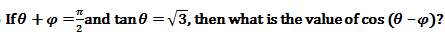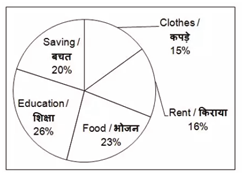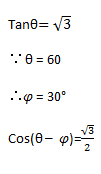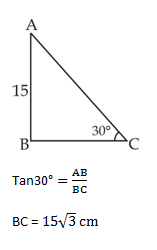# SSC Quantitative Aptitude Questions (Day-63)

Dear Aspirants, you can find the Quantitative Aptitude questions with detailed explanations for the SSC exams. Nowadays the competitive level of the exam has been increasing consistently. Due to the great demand for the government job, the level of the toughness reached greater. Candidates have to enhance the preparation process in order to drive in the right path. It doesn’t need to clear the prescribed cutoff. You must have to score good marks more than the cut off marks to get into the final provisional list. Here we have updating the Quantitative Aptitude questions with detailed explanations on a daily basis. You can practice with us and measure your level of preparation. According to that you can sculpt yourself in a proper way. SSC aspirants kindly make use of it and grab your success in your career.

Start Quiz

1)(a) 1/2

(b) √3/2

(c) 1

(d) 1/√2

2) If x and y are positive acute angles such that sin (2x + 3y) =
3/2 and cos (4x – 3y) = 3/2 , then what is the value of tan (6x – 3y)?

(a) 0

(b) 1

(c) 1/√3

(d) √3

3) If 3 cot θ = 4 cos θ, then what is the value of cos2θ?

(a) 2/16

(b) – 1/8

(c) 7/16

(d) 9/16

4) A person observes that the angle of elevation of the top of a pole of height 15 m is 30°. What is the distance (in metres) of the person from the pole?

(a) 15

(b) 15√3

(c) 15/√3

(d) 30

5) If cosec2 θ + cot2 θ = 7, then what is the value (in degrees) of θ?

(a) 15

(b) 30

(c) 45

(d) 60

Direction (6-10): The given pie chart shows the breakup (in percentage) of monthly expenditure of a person.6) If the total salary of the person is Rs 50000, then what will be the expenditure (in Rs) on Rent?

(a) 16000

(b) 12000

(c) 7000

(d) 8000

7) What is the central angle (in degrees) made by the sector of expenditure on Education?

(a) 93.6

(b) 88.2

(c) 84.3

(d) 95.2

8) The expenditure on food is how much percent more than the expenditure on Savings?

(a) 12.21

(b) 15

(c) 13.04

(d) 11

9) If the expenditure incurred on Clothes is Rs 3000, then what is the expenditure (in Rs) incurred on Education?

(a) 4300

(b) 5050

(c) 5200

(d) 5600

10) The central angle made by the sector of expenditure on Fuel is how much more (in degrees) than the central angle made by the sector of expenditure on Clothes, ifthe ratio of the expenditure on Fuel and Clothes is 4 : 3 respectively?

(a) 18

(b) 32

(c) 36

(d) 45x = 15

y = 10

tan (6x-3y) = tan(60) = √3

3cotθ  = 4 cosθ

¾ =  sinθ

Cos2θ = 1 – 2sin²θ

= 1 – 2 × 9/16

= -1/8Cosec²θ + cot²θ  = 7

Cosec²θ – cot²θ + 2cot²θ = 7

1 + 2cot²θ = 7

Cot²θ = 3

θ = 30°

Rent expenditure = 16%

Hence ⇒   16/100 × 50000

= 8000/-

∵ 1% → 3.6°

26% → 3.6 × 26

= 93.6°

Expenditure on food = 23%

Savings = 20%

% of expenditure on food

= (23-20) / 20 × 100

= 3/20 × 100

= 15%

If expenditure on clothes

15% ⇒ 3000

Then, expenditure on education

26% = 3000/15 × 26

= 5200

F/C = 4/3 = 20/15

Difference ⇒  5%

Angle for 5% = 5/100 × 360

= 18

 Check Here to View SSC CGL / CHSL 2021 Quantitative Aptitude Questions Day – 62 Day – 61 Day – 60